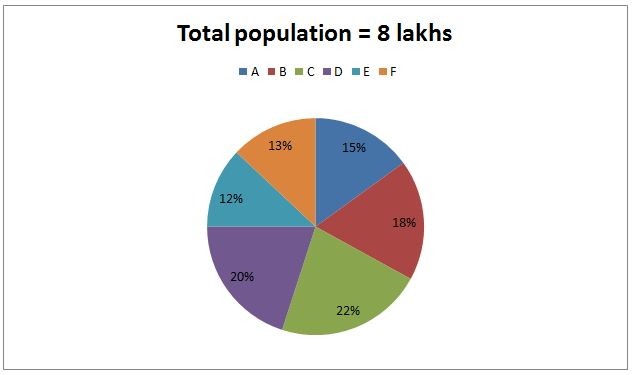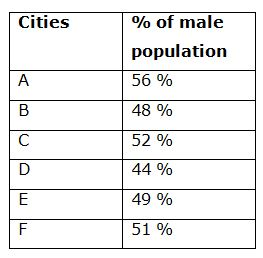# NIACL AO Prelims – Quantitative Aptitude Questions Day- 82

Dear Readers, Bank Exam Race for the Year 2019 is already started, To enrich your preparation here we have providing new series of Practice Questions on Quantitative Aptitude – Section. Candidates those who are preparing for NIACL AO Prelims 2019 Exams can practice these questions daily and make your preparation effective.

[WpProQuiz 4960]

1) Simple interest on a certain sum of money for 2 years at 6% per annum is half the compound interest on Rs. 15000 for 2 years at 10% per annum. The sum placed on simple interest is?

a) Rs. 18500

b) Rs. 21250

c) Rs. 13125

d) Rs. 15725

e) None of these

2) A box contains 5 red balls, 4 pink balls and 3 yellow balls. If 2 balls are drawn at randomly, then the probability of getting at least one ball is pink?

a) 19/33

b) 71/83

c) 57/75

d) 45/62

e) None of these

3) The sum of (3/5) of a number and 42 % of the same number is 255. What is the 54 % of the number?

a) 180

b) 225

c) 160

d) 135

e) None of these

4) The ratio of present ages of P and Q is 1: 3. The present age of P is 3 times the present age of R. Sum of the present age of P, Q and R is 65 years. Find the present age of Q?

a) 15 years

b) 45 years

c) 5 years

d) 60 years

e) None of these

5) A, B and C started a business by investing in the ratio of 2: 3: 4. After 4 months, A invested Rs. 10000 more and B withdraw Rs. 5000. Find the initial investment of C, if the ratio of share of A, B and C is 2: 2: 3?

a) Rs. 30000

b) Rs. 45000

c) Rs. 40000

d) Rs. 35000

e) None of these

Directions (6 – 10): Study the following information carefully and answer the given questions:

Following pie chart shows the total number of population in different cities in certain state and the table shows the percentage of male among them.6) The total female population of city A is what percentage of total female population of city C?

a) 62.5 %

b) 75 %

c) 56.25 %

d) 70.5 %

e) None of these

7) Find the ratio between the total population of city B to that of total male population of E and F together?

a) 142: 113

b) 166: 125

c) 247: 158

d) 200: 139

e) None of these

8) Find the difference between the total female population of city D to that of total male population of city B?

a) 18560

b) 20480

c) 16420

d) 17890

e) None of these

9) Find the total male population of city C, D and E together?

a) 256820

b) 289490

c) 208960

d) 234870

e) None of these

10) If the percentage of literate males and illiterate females of city D is 72 % and 34 % respectively, then find the difference between the literate males and literate females in city D?

a) 8448

b) 9256

c) 7042

d) 9564

e) 8640

Compound Interest:

15000*(10/100) = 1500

16500*(10/100) = 1650

C.I = 3150

S.I = (1/2)*C.I = 3150/2 = 1575

According to the question,

= > S.I = PNR/100

= > 1575 = P*2*6/100

= > P = (1575*100)/12

= > P = Rs. 13125

Total probability n(S) = 12C2

Required probability = 1 – P(none is pink)

Probability of getting none is pink balls,

P(E) = n(E)/n(S)

P(E) = 8C2/12C2 = 14/33

Required probability = 1 – P(none is pink)

= > 1 – (14/33)

= > 19/33

(3/5)x + (42/100)x = 255

(60x + 42x)/ 100 = 255

(102x/100) = 255

X = 255*(100/102) = 250

54 % of the number = 250*(54/100) = 135

The ratio of present ages of P and Q = 1: 3 (x, 3x)

P = 3R

R = x/3

P + Q + R = 65

X + 3x + (x/3) = 65

13x/3 = 65

13x = 65*3

X = 15

Present age of Q = 3x = 45 years

The share of A, B and C

= > [2x*4 + (2x + 10000)*8]: [3x*4 + (3x – 5000)*8]: [4x*12]

= > [24x + 80000]: [36x – 40000]: [48x]

According to the question,

(36x – 40000)/48x = (2/3)

36x – 40000 = 32x

4x = 40000

X = 10000

Initial investment of C = 4x = Rs. 40000

Directions (6-10):

The total female population of city A

= > 800000*(15/100)*(44/100)

= > 52800

The total female population of city C

= > 800000*(22/100)*(48/100)

= > 84480

Required % = (52800/84480)*100 = 62.5 %

The total population of city B

= > 800000*(18/100) = 144000

The total male population of E and F together

= > 800000*(12/100)*(49/100) + 800000*(13/100)*(51/100)

= > 47040 + 53040 = 100080

Required ratio = 144000: 100080 = 200: 139

The total female population of city D

= > 800000*(20/100)*(56/100)

= > 89600

The total male population of city B

= > 800000*(18/100)*(48/100)

= > 69120

Required difference = 89600 – 69120 = 20480

The total male population of city C, D and E together

= > 800000*(22/100)*(52/100) + 800000*(20/100)*(44/100) + 800000*(12/100)*(49/100)

= > 91520 + 70400 + 47040

= > 208960

Total male population in city D

= > 800000*(20/100)*(44/100) = 70400

Total literate males in city D

= > 70400*(72/100) = 50688

Total female population in city D

= > 800000*(20/100)*(56/100) = 89600

Total literate females in city D

= > 89600*(66/100) = 59136

Required difference = 59136 – 50688 = 8448